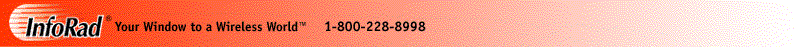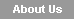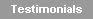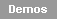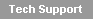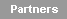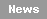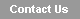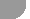FREE InfoRad® Wireless 30-day Trials

Please take a moment to register. Thank you!
Note: Items marked with an "*" are required fields.

```*First Name:

*Last Name:

Title:

Company name:

*E-mail address:

*Mailing address:

*City, State, Zip:

*Phone Number: FAX Number:

Sales Rep Code:

*Demo to Download:```
```Industry:

N/A Healthcare Commercial/Industrial Government Public Safety
Education Communications Application Development Personal```
```Company (Number of employees)

N/A 0-99  100-499 500+ ```
```Internet Access:

N/A Dial-up Network DSL Cable ISDN Other ```
```Network OS Used:

N/A Windows 2012 Windows 2008 Windows 2003 Novell Other ```
```Desktop OS Used:

N/A Windows 10 Windows 8 Windows 7 Windows XP Other ```
```How did you find us:

N/A Current InfoRad customer Wireless Carrier Web search E-mail Post Card
Internet Ad Print Ad or story Colleague Friend Family Yellow Pages Other ```
```What type of wireless device do you use:

N/A One-way pager Two-way pager Phone Tablet Blackberry Other ```
```Who is your wireless provider:
<!--

if (value == null)
{
document.write("");
}
else if (value == "erad019")
{
document.write("<input type=\"radio\" value=\"Advantage Comm\" name=\"WirelessProvider\" CHECKED>Advantage Comm ");
}
else if (value == "erad025")
{
document.write("<input type=\"radio\" value=\"AllPage\" name=\"WirelessProvider\" CHECKED>AllPage ");
}
else if (value == "erad024")
{
document.write("<input type=\"radio\" value=\"AllPage\" name=\"WirelessProvider\" CHECKED>AllPage ");
}
else if (value == "erad021")
{
document.write("<input type=\"radio\" value=\"Cook Paging\" name=\"WirelessProvider\" CHECKED>Cook Paging ");
}
else if (value == "erad022")
{
document.write("<input type=\"radio\" value=\"Teletouch\" name=\"WirelessProvider\" CHECKED>Teletouch ");
}
else if (value == "erad023")
{
document.write("<input type=\"radio\" value=\"PageOne NW\" name=\"WirelessProvider\" CHECKED>PageOne NW ");
}
else if (value == "erad028")
{
document.write("<input type=\"radio\" value=\"Baystar Comm.\" name=\"WirelessProvider\" CHECKED>Baystar Comm. ");
}
else if (value == "erad026")
{
document.write("<input type=\"radio\" value=\"Salisbury Comm.\" name=\"WirelessProvider\" CHECKED>Salisbury Comm. ");
}
else if (value == "erad036")
{
document.write("<input type=\"radio\" value=\"N/A\" name=\"WirelessProvider\" CHECKED>N/A ");
}
else if (value == "erad034")
{
document.write("<input type=\"radio\" value=\"ALLTEL\" name=\"WirelessProvider\" CHECKED>ALLTEL ");
}
else if (value == "erad030")
{
document.write("<input type=\"radio\" value=\"Network Services\" name=\"WirelessProvider\" CHECKED>Network Services ");
}
else if (value == "erad033")
{
document.write("<input type=\"radio\" value=\"Indiana Paging Network\" name=\"WirelessProvider\" CHECKED>Indiana Paging Network ");
}
else if (value == "erad041")
{
document.write("<input type=\"radio\" value=\"CANPAGE\" name=\"WirelessProvider\" CHECKED>CANPAGE ");
}
else if (value == "erad027")
{
document.write("");
}
else if (value == "erad042")
{
document.write("<input type=\"radio\" value=\"N/A\" name=\"WirelessProvider\" CHECKED>N/A ");
}
else if (value == "erad038")
{
document.write("<input type=\"radio\" value=\"ValuePage\" name=\"WirelessProvider\" CHECKED>ValuePage ");
}
else if (value == "erad031")
{
document.write("<input type=\"radio\" value=\"Verizon\" name=\"WirelessProvider\" CHECKED>Verizon ");
}
else if (value == "erad042A")
{
document.write("<input type=\"radio\" value=\"N/A\" name=\"WirelessProvider\" CHECKED>N/A ");
}
else if (value == "erad000C")
{
document.write("<input type=\"radio\" value=\"N/A\" name=\"WirelessProvider\" CHECKED>N/A ");
}
else if (value == "erad042B")
{
document.write("<input type=\"radio\" value=\"N/A\" name=\"WirelessProvider\" CHECKED>N/A ");
}
else if (value == "erad042C")
{
document.write("<input type=\"radio\" value=\"N/A\" name=\"WirelessProvider\" CHECKED>N/A ");
}
else if (value == "erad042D")
{
document.write("<input type=\"radio\" value=\"N/A\" name=\"WirelessProvider\" CHECKED>N/A ");
}
else if (value == "erad042E")
{
document.write("<input type=\"radio\" value=\"N/A\" name=\"WirelessProvider\" CHECKED>N/A ");
}
else if (value == "erad042F")
{
document.write("<input type=\"radio\" value=\"N/A\" name=\"WirelessProvider\" CHECKED>N/A ");
}
else if (value == "erad029")
{
document.write("<input type=\"radio\" value=\"SouthernLINC\" name=\"WirelessProvider\" CHECKED>SouthernLINC ");
}
else if (value == "erad046")
{
document.write("<input type=\"radio\" value=\"UCOM Paging\" name=\"WirelessProvider\" CHECKED>UCOM Paging ");
}
else if (value == "erad047")
{
document.write("<input type=\"radio\" value=\"Advanced RF Comm.\" name=\"WirelessProvider\" CHECKED>Advanced RF Comm. ");
}
else if (value == "erad048")
{
document.write("<input type=\"radio\" value=\"Bailey's Comm.\" name=\"WirelessProvider\" CHECKED>Bailey's Comm. ");
}
else if (value == "erad049")
{
document.write("<input type=\"radio\" value=\"Satellink\" name=\"WirelessProvider\" CHECKED>Satellink ");
}
else if (value == "erad050")
{
document.write("<input type=\"radio\" value=\"ProPage\" name=\"WirelessProvider\" CHECKED>ProPage ");
}
else if (value == "erad042G")
{
document.write("<input type=\"radio\" value=\"N/A\" name=\"WirelessProvider\" CHECKED>N/A ");
}
else if (value == "erad042H")
{
document.write("<input type=\"radio\" value=\"N/A\" name=\"WirelessProvider\" CHECKED>N/A ");
}
else if (value == "erad042I")
{
document.write("<input type=\"radio\" value=\"N/A\" name=\"WirelessProvider\" CHECKED>N/A ");
}
else if (value == "erad042J")
{
document.write("<input type=\"radio\" value=\"N/A\" name=\"WirelessProvider\" CHECKED>N/A ");
}
else if (value == "erad042K")
{
document.write("<input type=\"radio\" value=\"N/A\" name=\"WirelessProvider\" CHECKED>N/A ");
}
else if (value == "erad053")
{
document.write("<input type=\"radio\" value=\"Roger's Two-Way\" name=\"WirelessProvider\" CHECKED>Roger's Two-Way ");
}
else if (value == "erad045")
{
document.write("<input type=\"radio\" value=\"Mobilfone\" name=\"WirelessProvider\" CHECKED>Mobilfone ");
}
else if (value == "erad054")
{
document.write("<input type=\"radio\" value=\"Radio Comm Paging\" name=\"WirelessProvider\" CHECKED>Radio Comm Paging ");
}
else if (value == "erad057")
{
document.write("<input type=\"radio\" value=\"American Messaging\" name=\"WirelessProvider\" CHECKED>American Messaging ");
}
else if (value == "erad058")
{
document.write("<input type=\"radio\" value=\"WaveWare\" name=\"WirelessProvider\" CHECKED>WaveWare ");
}
else if (value == "erad059")
{
document.write("<input type=\"radio\" value=\"Pagenet Canada\" name=\"WirelessProvider\" CHECKED>Pagenet Canada ");
}
else if (value == "erad060")
{
document.write("<input type=\"radio\" value=\"US Cellular\" name=\"WirelessProvider\" CHECKED>US Cellular ");
}
else if (value == "erad061")
{
document.write("<input type=\"radio\" value=\"USA Mobility\" name=\"WirelessProvider\" CHECKED>USA Mobility ");
}
else if (value == "erad064")
{
document.write("<input type=\"radio\" value=\"Gulf State Wireless\" name=\"WirelessProvider\" CHECKED>Gulf State Wireless ");
}
else if (value == "erad500")
{
document.write("<input type=\"radio\" value=\"HandyPage\" name=\"WirelessProvider\" CHECKED>HandyPage ");
}
else if (value == "erad083")
{
document.write("<input type=\"radio\" value=\"Page 1\" name=\"WirelessProvider\" CHECKED>Page 1 ");
}
else if (value == "erad084")
{
document.write("<input type=\"radio\" value=\"RCV\" name=\"WirelessProvider\" CHECKED>RCV ");
}
else if (value == "erad104")
{
document.write("<input type=\"radio\" value=\"Midwest Paging\" name=\"WirelessProvider\" CHECKED>Midwest Paging ");
}
else if (value == "erad106")
{
document.write("<input type=\"radio\" value=\"Rinkers Communications\" name=\"WirelessProvider\" CHECKED>Rinkers Communications ");
}
else if (value == "erad112")
{
document.write("<input type=\"radio\" value=\"Contact Wireless\" name=\"WirelessProvider\" CHECKED>Contact Wireless ");
}
else if (value == "erad901")
{
document.write("<input type=\"radio\" value=\"American Messaging\" name=\"WirelessProvider\" CHECKED>American Messaging ");
}
else
{
document.write("</p>");
}

-->ALLTEL American Messaging AT&T Sprint PCS T-Mobile USA Mobility|SPOK Verizon Other Paging Carrier Other Cellular/PCS Carrier ```
```If Other, please enter your Wireless Provider:
```
```Would you like to be on our e-mail list:

All Mailings New Products Sales Specials Newsletters No Thanks   ```
`  `
```NOTE: If download option does not appear, you may need to disable your web browser pop-up blocker to download.
Example: Internet Explorer 11 - Settings menu - Internet options - Privacy - Pop-up Blocker option.
Temporarily disable; or in Settings add www.inforad.com to allowed sites.
Alternately, an email has been sent to your email address with direct download links.```
` `
` `
` `
```

if (value == null)
{
var SRCVAL="demos";
}
else if (value == "erad019")
{
var SRCVAL="Advantage Comm";
}
else if (value == "erad025")
{
var SRCVAL="AllPage of Houston";
}
else if (value == "erad024")
{
var SRCVAL="Allpage of Mobile";
}
else if (value == "erad021")
{
var SRCVAL="Cook Paging";
}
else if (value == "erad022")
{
var SRCVAL="Teletouch";
}
else if (value == "erad023")
{
var SRCVAL="PageOne NW";
}
else if (value == "erad028")
{
var SRCVAL="Baystar Communications";
}
else if (value == "erad026")
{
var SRCVAL="Salisbury Communications";
}
else if (value == "erad036")
{
var SRCVAL="Paging Information Resource";
}
else if (value == "erad034")
{
var SRCVAL="ALLTEL";
}
else if (value == "erad030")
{
var SRCVAL="Network Services";
}
else if (value == "erad033")
{
var SRCVAL="Indiana Paging Network";
}
else if (value == "erad041")
{
var SRCVAL="CANPAGE";
}
else if (value == "erad027")
{
var SRCVAL="Arch Wireless";
}
else if (value == "erad042")
{
var SRCVAL="Google 042";
}
else if (value == "erad038")
{
var SRCVAL="ValuePage";
}
else if (value == "erad031")
{
var SRCVAL="Verizon Wireless";
}
else if (value == "erad042A")
{
var SRCVAL="Google 042A";
}
else if (value == "erad000C")
{
var SRCVAL="000C";
}
else if (value == "erad042B")
{
var SRCVAL="Google 042B";
}
else if (value == "erad042C")
{
var SRCVAL="Google 042C";
}
else if (value == "erad042D")
{
var SRCVAL="Google 042D";
}
else if (value == "erad042E")
{
var SRCVAL="Google 042E";
}
else if (value == "erad042F")
{
var SRCVAL="Google 042F";
}
else if (value == "erad029")
{
var SRCVAL="SouthernLINC";
}
else if (value == "erad046")
{
var SRCVAL="UCOM Paging";
}
else if (value == "erad047")
{
var SRCVAL="Advanced RF Comm";
}
else if (value == "erad048")
{
var SRCVAL="Bailey's Communications";
}
else if (value == "erad049")
{
var SRCVAL="Satellink";
}
else if (value == "erad050")
{
var SRCVAL="ProPage";
}
else if (value == "erad042G")
{
var SRCVAL="Google 042G";
}
else if (value == "erad042H")
{
var SRCVAL="Google 042H";
}
else if (value == "erad042I")
{
var SRCVAL="Google 042I";
}
else if (value == "erad042J")
{
var SRCVAL="Google 042J";
}
else if (value == "erad042K")
{
var SRCVAL="Google 042K";
}
else if (value == "erad053")
{
var SRCVAL="Roger's Two-Way";
}
else if (value == "erad045")
{
var SRCVAL="Mobilfone";
}
else if (value == "erad054")
{
var SRCVAL="Radio Comm Paging";
}
else if (value == "erad057")
{
var SRCVAL="American Messaging";
}
else if (value == "erad058")
{
var SRCVAL="WaveWare";
}
else if (value == "erad059")
{
var SRCVAL="Pagenet Canada";
}
else if (value == "erad060")
{
var SRCVAL="US Cellular";
}
else if (value == "erad061")
{
var SRCVAL="USA Mobility";
}
else if (value == "erad064")
{
var SRCVAL="Gulf State Wireless";
}
else if (value == "erad500")
{
var SRCVAL="HandyPage";
}
else if (value == "erad035")
{
var SRCVAL="Zetron";
}
else if (value == "erad083")
{
var SRCVAL="Page1WV";
}
else if (value == "erad084")
{
var SRCVAL="RCV";
}
else if (value == "erad104")
{
var SRCVAL="Midwest Paging";
}
else if (value == "erad106")
{
var SRCVAL="Rinkers Communications";
}
else if (value == "erad112")
{
var SRCVAL="Contact Wireless";
}
else if (value == "erad901")
{
var SRCVAL="AlphaCast";
}
else
{
var SRCVAL="demos";
}

Today is:

document.register.date.value=todayStr()

Source:

document.register.source.value=SRCVAL

Copyright © 2000 - 2016 InfoRad, Inc.
All Rights Reserved
Makers of AlphaPage® Wireless Messaging Products since 1985.
```